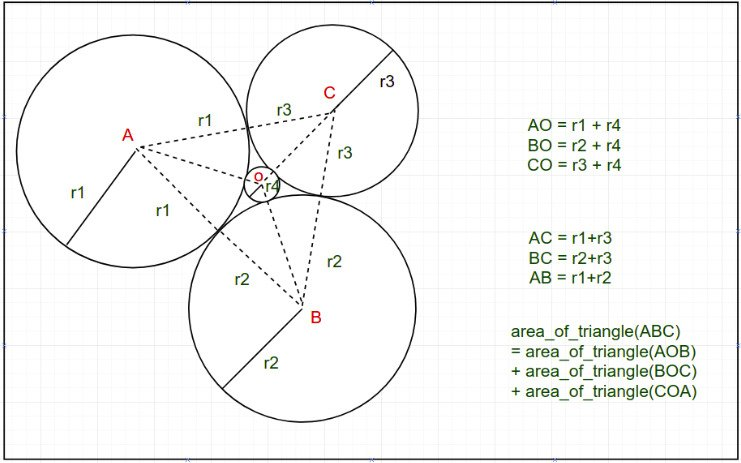# Descartes’ Circle Theorem with implementation

In geometry, Descartes’ theorem states that for every four mutually tangent circles, the radii of the circles satisfy a certain quadratic equation. One can construct a fourth circle tangent to three given, mutually tangent circles.
Descartes’ Circle Theorem helps us to find the radius of a circle when there are 4 circles with positive integer radius r1, r2, r3 and r4 as shown in the figure below. It finds the radius r4 of the circle formed by three circles of radius r1, r2, r3 as shown in the image below.
(Note that the circles in the picture below are tangent to each other.)Examples:

Input: r1 = 1, r2 = 1, r3 = 1
Output: 0.154701

Input: r1 =23, r2 = 46, r3 = 69
Output: 6.000000

The theorem says that the reciprocals of radii, or “curvatures”, of these circlessatisfies the following relation:One of the circles can be enclosed among all the remaining three, as in the figure, in which case the corresponding curvaturehere is considered negative and the above relation still holds.
Ifare known, one can solve for k4,On solving the above equation we get the radius of the fourth circle. The formula for finding the fourth circle’s radius is:Thus if r1, r2, and r3 are known, r4 can easily be calculated using the above formula.

Below is the implementation of the above approach:

## CPP

 // C++ implementation of the // above formulae #include  using namespace std;   // Function to find the fourth circle's // when three radius are given double findRadius(double r1, double r2, double r3) {     double r4 = (r1 * r2 * r3)                 / (r1 * r2 + r2 * r3                    + r1 * r3 + 2.0 * sqrt(r1 * r2 * r3 * (r1 + r2 + r3)));       return r4; }   // Driver code int main() {     // Radius of three circles     double r1 = 1;     double r2 = 1;     double r3 = 1;       // Calculation of r4 using formula given above     double r4 = findRadius(r1, r2, r3);       cout << "The radius of fourth circle: " << r4;     return 0; }

## Java

 /*package whatever //do not write package name here */ // Java implementation of the // above formulae import java.io.*;   class GFG {             // Function to find the fourth circle's         // when three radius are given         static double findRadius(double r1, double r2, double r3)         {         double r4 = (r1 * r2 * r3)                 / (r1 * r2 + r2 * r3                    + r1 * r3 + 2.0 * Math.sqrt(r1 * r2 * r3 * (r1 + r2 + r3)));           return r4;         }           // Driver code     public static void main (String[] args)     {                 // Radius of three circles     double r1 = 1;     double r2 = 1;     double r3 = 1;       // Calculation of r4 using formula given above     double r4 = findRadius(r1, r2, r3);         System.out.println("The radius of fourth circle: " + r4);     } }   // This code is contributed by CoderSaty.

## Python3

 # Python 3 implementation of the # above formulae   from math import sqrt   # Function to find the fourth circle's # when three radius are given def findRadius(r1, r2, r3):     r4 = (r1 * r2 * r3) / (r1 * r2 + r2 * r3 + r1 * r3 + 2.0 * sqrt(r1 * r2 * r3 * (r1 + r2 + r3)))       return r4   # Driver code if __name__ == '__main__':         # Radius of three circles     r1 = 1     r2 = 1     r3 = 1       # Calculation of r4 using formula given above     r4 = findRadius(r1, r2, r3)       print("The radius of fourth circle:",r4)           # This code is contributed by SURENDRA_GANGWAR.

## C#

 // C# implementation of the // above formulae using System;   class GFG {     // Function to find the fourth circle's   // when three radius are given   static double findRadius(double r1, double r2, double r3)   {     double r4 = (r1 * r2 * r3)       / (r1 * r2 + r2 * r3          + r1 * r3 + 2.0 * Math.Sqrt(r1 * r2 * r3 * (r1 + r2 + r3)));       return r4;   }     // Driver code   public static void Main (String[] args)   {       // Radius of three circles     double r1 = 1;     double r2 = 1;     double r3 = 1;       // Calculation of r4 using formula given above     double r4 = Math.Round(findRadius(r1, r2, r3),6);       Console.Write("The radius of fourth circle: " + r4);   } }   // This code is contributed by shivanisinghss2110

## Javascript

 

Output

The radius of fourth circle: 0.154701

Time complexity: O(log(r1 * r2 * r3 * (r1 + r2 + r3)))
Auxiliary space: O(1)

Feeling lost in the world of random DSA topics, wasting time without progress? It's time for a change! Join our DSA course, where we'll guide you on an exciting journey to master DSA efficiently and on schedule.
Ready to dive in? Explore our Free Demo Content and join our DSA course, trusted by over 100,000 geeks!

Previous
Next